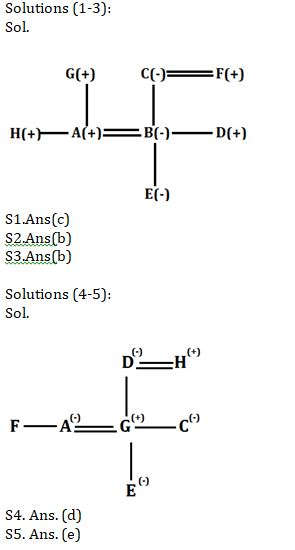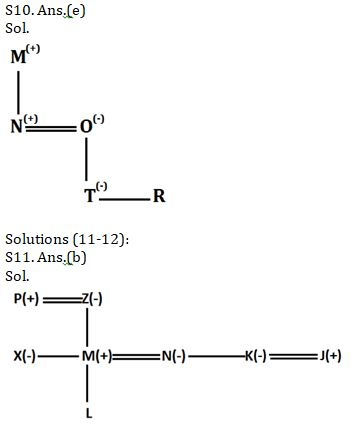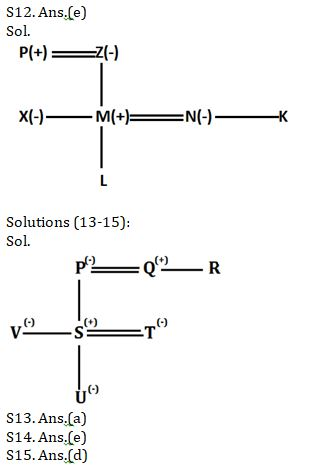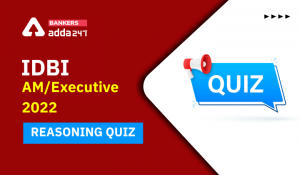Latest Banking jobs   »   Reasoning Ability Quiz For SBI Clerk...

# Reasoning Ability Quiz For SBI Clerk Prelims 2021- 14th May

Direction (1-3): Study the following information carefully and answer the given questions.
There are eight members in a family i.e. A, B, C, D, E, F, G and H. There are three females and two married couples. A is son in law of C. B is daughter in law of G, who is father of H. H is unmarried. D is son of C. C married to F, who is grandfather of E. E is niece of H who is brother of A.

Q1. Who among the following is brother-in-law of D?
(a) G
(b) C
(c) A
(d) B
(e) None of these

Q2. Who among the following is brother in law of B?
(a) A
(b) H
(c) D
(d) G
(e) None of these

Q3. Who among the following is father of D?
(a) E
(b) F
(c) A
(d) H
(e) None of these

Directions (4-5): Read the following information carefully and answer the given questions.
There are eight people in a family, all of them are going for vacation. There are two married couple in the family. C is the mother of B. E is the daughter of A, who is the sister of F. C is the only sister of G. H is father of C. D is the mother of C. A is the wife of G.

Q4. Who among the following is grandfather of E?
(a) B
(b) G
(c) C
(d) H
(e) None of these

Q5. How F is related to G?
(a) Sister-in-law
(b) Mother
(c) Son
(d) Brother
(e) Cannot be determine

Q6. In a row of boys, Ashu is 18th from the right end and Yatin is 35th from the left end. If in this row Yatin is Fifteenth from the right then what is the position of Ashu from the left?
(a) 31
(b) 32
(c) 30
(d) 33
(e) None of these

Direction (7-8): Study the following information carefully and answer the given questions:
Five persons A, B, C, D and E travel in a car, each of them has different weight. More than two persons are lighter than D. B is heavier than A but lighter than E. No one lighter than C. D is not the heaviest person.

Q7. Who among the following is heaviest person?
(a) C
(b) D
(c) E
(d) A
(e) B

Q8. If weight of third lightest person is 58kg, what is possible weight of A?
(a) 60kg
(b) 75kg
(c) 62kg
(d) 55kg
(e) 70kg

Q9. Introducing a man, a woman says, “He is the son-in-law of the wife of my father and I am the only child of my parents”. How is the man related to the woman?
(a) Nephew
(b) Husband
(c) Brother-in-law
(d) Cousin
(e) Mother

Q10. If ‘A + B’ means ‘A is father of B’, ‘A ÷ B’ means ‘A is mother of B’ , ‘A × B’ means ‘A is husband of B’, ‘A – B’ means ‘A is sister of B’, then in the given expression how R is related to M? ‘M+N×O÷T−R’
(a)Grandfather
(b)Grandson
(c) Granddaughter
(d) Son
(e) Cannot be determined

Directions (11-12): Study the information carefully and answer the questions given below.
P is father of M who is brother in law of K. N is daughter in law of Z who is grandmother of L. P has only two children one son and one daughter. X is sister in law of N. P is father in law of N. K is unmarried.

Q11. If K is wife of J then what could be the relation of J with respect to N?
(a) Brother
(b) Brother in law
(c) Uncle
(d) Cannot be determined
(e) None of these.

Q12. What is relation of L with respect to X?
(a) Nephew
(b) Niece
(c) Son
(d) Daughter
(e) Cannot be determined

Direction (13-15): Study the following information carefully to answer the given questions:
P, Q, R, S, U, V and T are the members of a family. There are three generation in this family. P is mother in law of T. V is sister of S who is nephew of R. U is granddaughter of Q. T is not married to V. T is a married woman. R is not sibling of P.

Q13. Who among the following is niece of V?
(a) U
(b) Q
(c) R
(d) T
(e) None of these

Q14. How R is related to V?
(a) Niece
(b) Father
(c) Aunt
(d) Uncle
(e) Cannot be determine

Q15. Who among the following is mother of U?
(a) V
(b) R
(c) P
(d) T
(e) None of these

ractice More Questions of Reasoning for Competitive Exams:

###### SBI Clerk Study Plan 2021

SolutionsS6. Ans(b)
Solution:
Clearly, Ashu is 18th from the right end and
Yatin is 35th from the left end and 15th from the right end of the row
So, number of boys in the row = (35 -1 + 15) = 49
Now, Ashu is 18th from the right
Ashu from left end= 49- (18-1)=32
Hence, Ashu is 32th from the left end of the row.

Solutions (7-8):
Sol. E > D > B > A > C
S7. Ans.(c)
S8. Ans.(d)

S9 Ans.(b)#### Congratulations!Incorrect details? Fill the form again here

•Reasoning Ability Quiz For IBPS RRB PO C...
•Reasoning Ability Quiz For IBPS Clerk Pr...
•Reasoning Ability Quiz For IBPS RRB PO P...
•Reasoning Ability Quiz For IDBI AM/Execu...
•Reasoning Ability Quiz For IBPS RRB PO P...
•Reasoning Ability Quiz For IDBI AM/Execu...